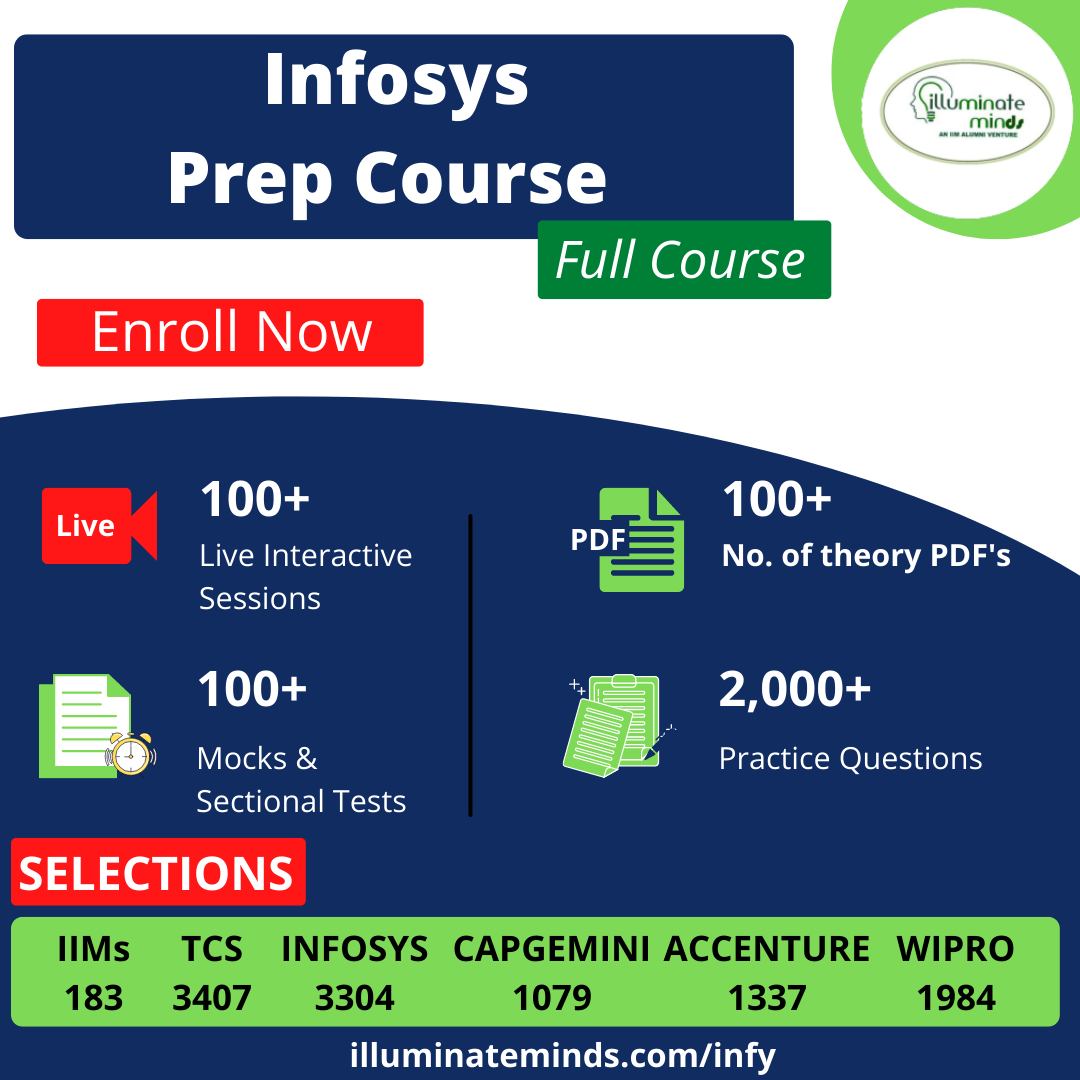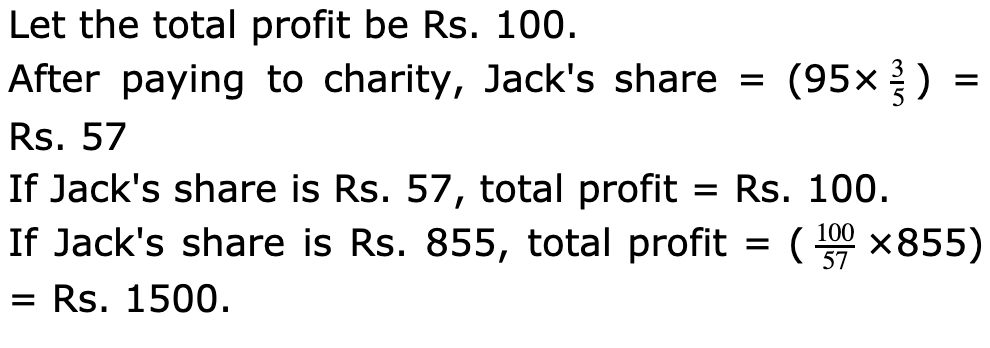HCL Quants Questions

## HCL Quants QuestionsJobs By Batch
Jobs By Location
Jobs By Degree
Jobs By Branch
IT Jobs
Internships
Govt. Jobs• ##### Original Questions of IT Companies| All Topics### Quiz Begins Here

Q #1
:

#### Quants Question

Directions: Read the following information to answer the question.

Jack and Jenny invest in a business in the ratio 3:2. If 5% of the total profit goes to charity and Jack's share is Rs. 855, the total profit is:
+Q #2
:

#### Quants Question

Directions: Read the following information to answer the question.

Shalini, Mahesh and Suresh enter into a partnership and their shares are in the ratio 1/2 : 1/3 : 1/4. After 2 months, Shalini withdraws half of his capital and after 10 months, a profit of Rs. 378 is divided among them. What is Mahesh's share?
+

Ratio of initial investments = 1/2 : 1/3 : 1/4 = 6 : 4 : 3.

Let their initial investments be 6x, 2x and 3x respectively.

Shalini : Mahesh : Suresh = (6x * 2 + 3x * 10) : (4x * 12) : (3x * 12) = 42 : 48 : 36 = 7 : 8 : 6.

Mahesh's share = Rs. (378*8/21) = Rs. 144

Q #3
:

#### Quants Question

Directions: Read the following information to answer the question.

What is the maximum percentage discount that a merchant can offer on her Marked Price so that she ends up selling at no profit or loss, if she had initially marked her goods up by 50%?
+

The merchant had initially marked her goods up by 50%.

Let us assume that her cost price of the goods to be \$ 100.

Therefore, a 50% mark up would have resulted in her marked price being \$100 + 50% of \$100 = \$100 + \$50 = \$150.

The question states that she finally sells the product at no profit or loss. This essentially, means that she sells the product at cost price, which in this case would be \$100.

Therefore, she had offered a discount of \$50 on her marked price of \$150.

Hence, the % discount offered by her = 50/150 * 100 = 33.33%

Q #4
:

#### Quants Question

Directions: Read the following information to answer the question.

*p is the least integer greater that p

@ p is the largest integer less than p

# (p, q) = Min (*p,@q)

What is the value of *4.5 - @3 + *4 + *7.2 - @2?

+

Given expression = 5 � 2 + 5 + 8 � 1 = 15

Q #5
:

#### Quants Question

Directions: Read the following information to answer the question.

If 5 geometric mean are inserted between 2 &128 , then find the value of 4th geometric mean .
+

Solution :

If we consider a=2 , ar6 = 128 , then r6 = 64 -> r =2 .

Therefore 4th geometric mean = ar4 = 2^5 = 32